Probability distribution

Chapter 13 Class 12 Probability (Term 2)
Concept wise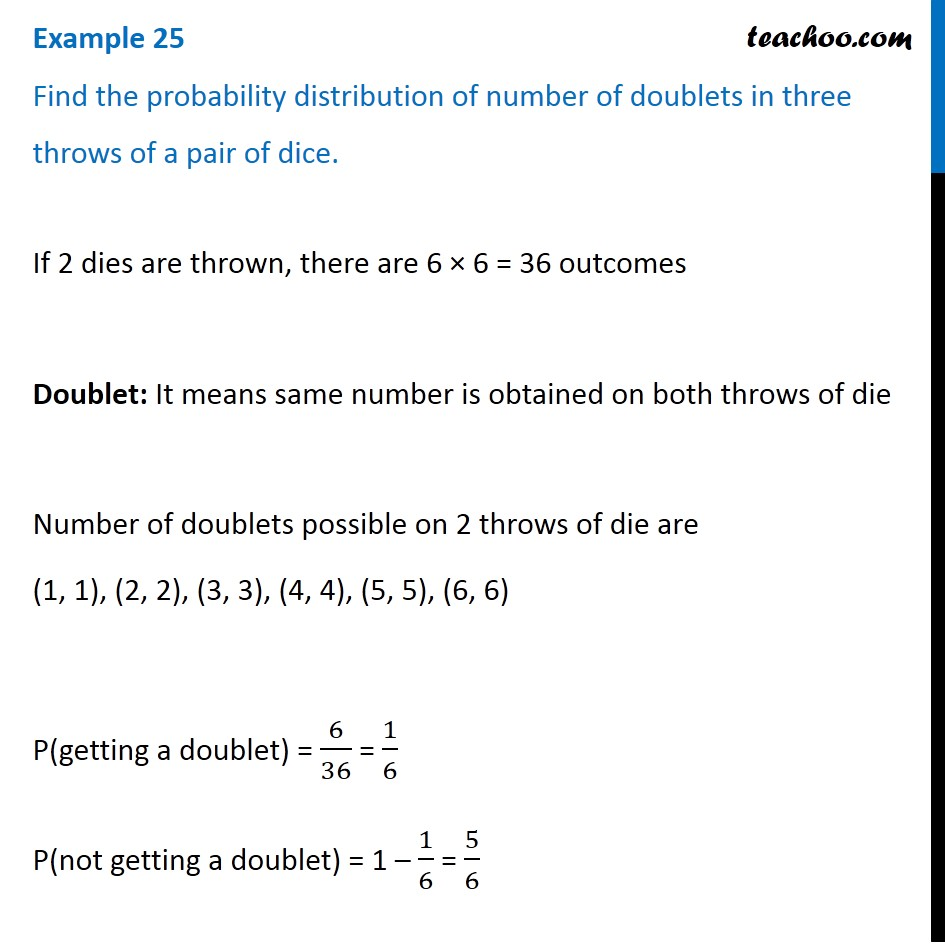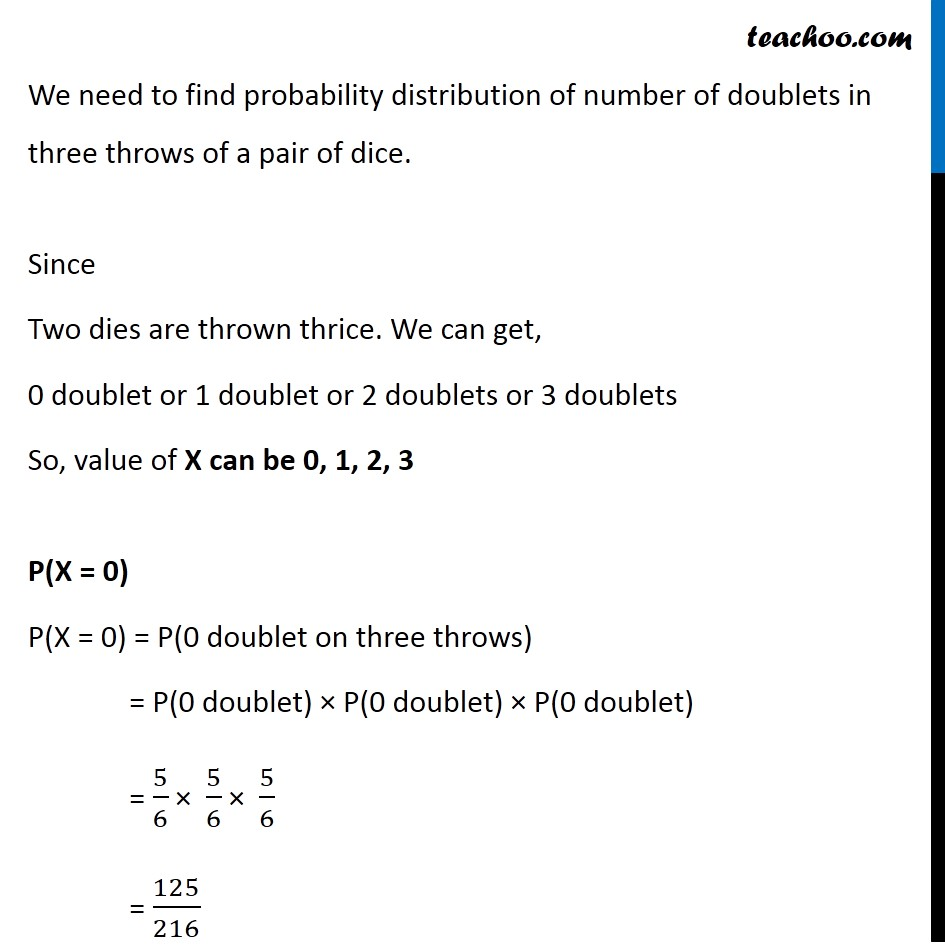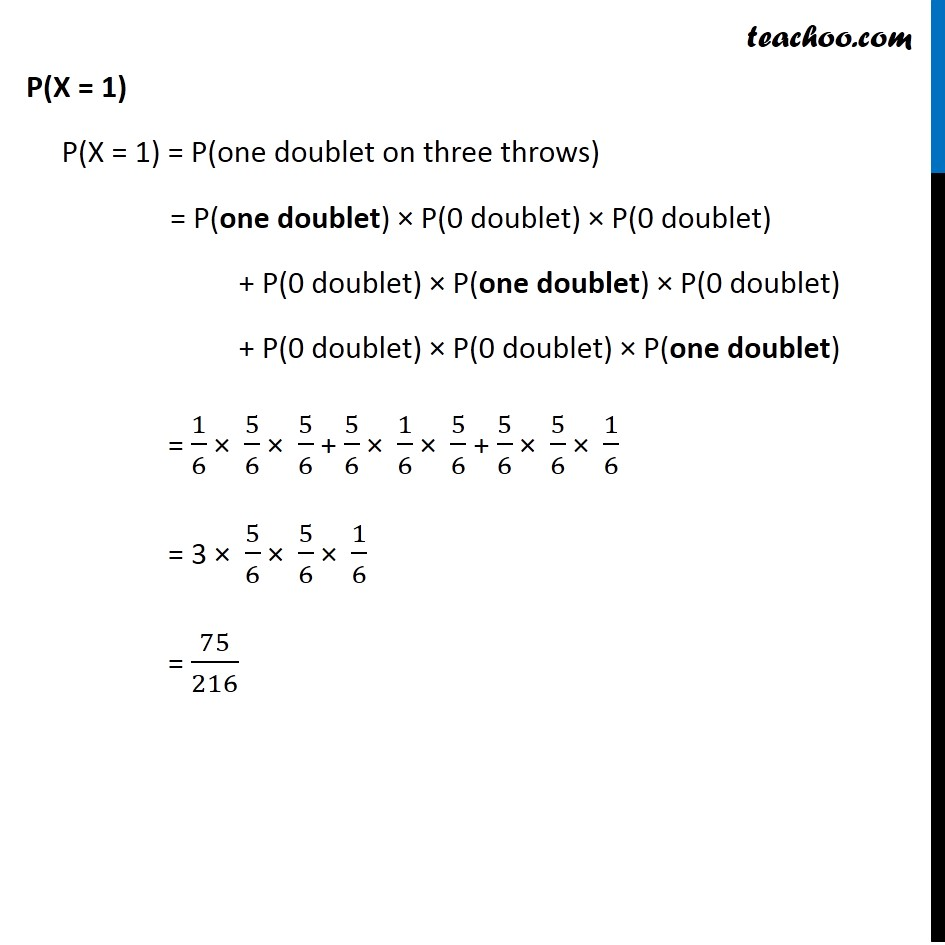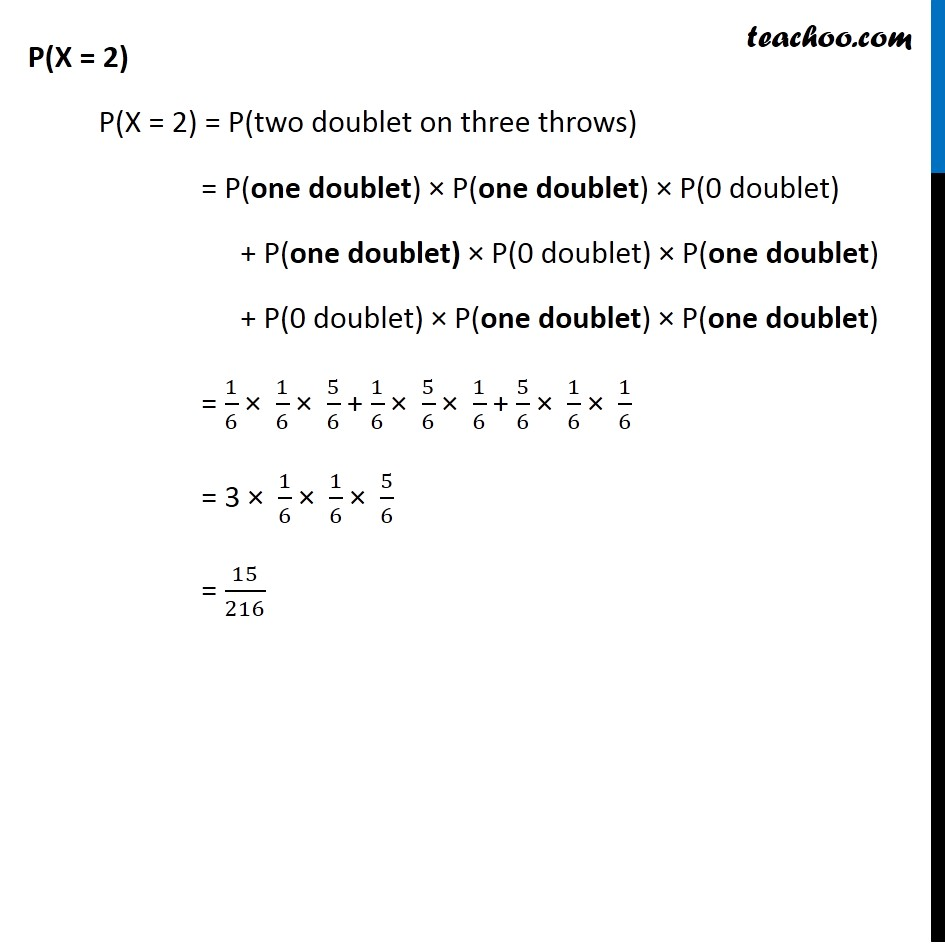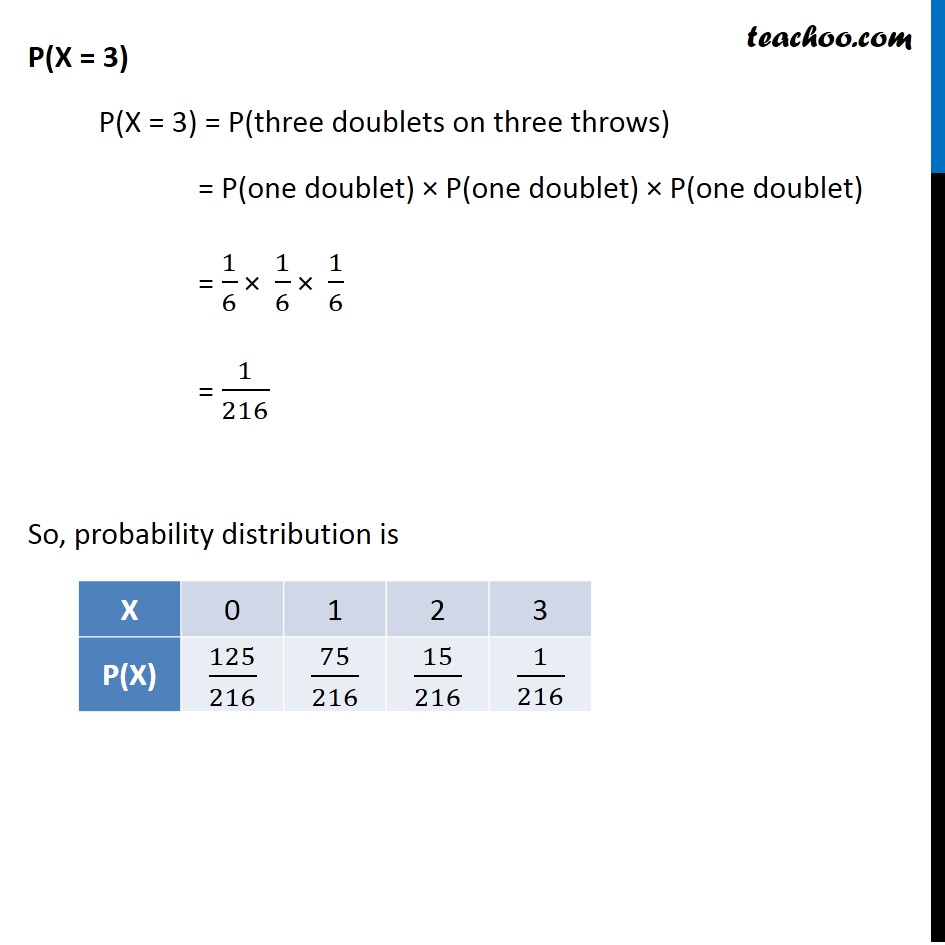### Transcript

Example 25 Find the probability distribution of number of doublets in three throws of a pair of dice. If 2 dies are thrown, there are 6 × 6 = 36 outcomes Doublet: It means same number is obtained on both throws of die Number of doublets possible on 2 throws of die are (1, 1), (2, 2), (3, 3), (4, 4), (5, 5), (6, 6) P(getting a doublet) = 6/36 = 1/6 P(not getting a doublet) = 1 – 1/6 = 5/6 We need to find probability distribution of number of doublets in three throws of a pair of dice. Since Two dies are thrown thrice. We can get, 0 doublet or 1 doublet or 2 doublets or 3 doublets So, value of X can be 0, 1, 2, 3 P(X = 0) P(X = 0) = P(0 doublet on three throws) = P(0 doublet) × P(0 doublet) × P(0 doublet) = 5/6 × 5/6 × 5/6 = 125/216 P(X = 1) P(X = 1) = P(one doublet on three throws) = P(one doublet) × P(0 doublet) × P(0 doublet) + P(0 doublet) × P(one doublet) × P(0 doublet) + P(0 doublet) × P(0 doublet) × P(one doublet) = 1/6 × 5/6 × 5/6 + 5/6 × 1/6 × 5/6 + 5/6 × 5/6 × 1/6 = 3 × 5/6 × 5/6 × 1/6 = 75/216 P(X = 2) P(X = 2) = P(two doublet on three throws) = P(one doublet) × P(one doublet) × P(0 doublet) + P(one doublet) × P(0 doublet) × P(one doublet) + P(0 doublet) × P(one doublet) × P(one doublet) = 1/6 × 1/6 × 5/6 + 1/6 × 5/6 × 1/6 + 5/6 × 1/6 × 1/6 = 3 × 1/6 × 1/6 × 5/6 = 15/216 P(X = 3) P(X = 3) = P(three doublets on three throws) = P(one doublet) × P(one doublet) × P(one doublet) = 1/6 × 1/6 × 1/6 = 1/216 So, probability distribution is